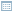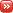• ## Bayesian Statistics in Layman's Terms

Posted by Konstantin 09.01.2016

This is a (slightly updated) repost of my quora answer to the corresponding question.

There are many ways in which smart people tend to explain Bayesian statistics and contrast it with a "non-Bayesian" one. One usually highlights that the primary concept of a Bayesian approach is the the desire to model everything as a probability distribution. Once this is fact is clear, many smart people would proceed to claim that this is, in fact, what fundamentally sets Bayesian statistics aside from the "classical" one. However, I feel that this kind of explanation is somewhat incomplete. It is not like classical statisticians do not use complete probability distributions. The difference is in general somewhat more subtle and philosophical.

Consider the question "what is your height?". For a classical statistician there exists some abstract "true answer", say "180cm", which is a fixed number - your one and only height. The problem is, of course, you do not know this number because every measurement is slightly different, so the classical statistician will add that "there is a normally-distributed measurement error". In the world of a pure Bayesian there are almost no "fixed numbers" - everything is a probability distribution, and so is your height! That is, a Bayesian should say that "your height is a Normal distribution centered around 180cm".

Note that from the mathematical perspective there is no difference between the two representations - in both cases the number 180cm is mentioned, and the normal distribution. However, from a philosophical, syntactical, methodological and "mental" perspectives this tends to have serious implications, and there has been historically a kind of an ongoing intellectual feud between the statisticians who lend more towards the first or the second approach (it is somewhat resemblant of how there is a divide among the physicists with regard to their support of the Copenhagen interpretation of quantum mechanics).

One of the implications of denying the fact that things in the world are mostly fixed (and are all pure distributions instead) is that you may not use many of the common sense inference methods directly. What is my height if I stand on a chair? "Well, it is your height plus the height of a chair", a classical statistician would say. He can keep in mind the measurement errors, if necessary, but those could be dealt with later. In the Bayesian world heights are not numbers, so the procedure of adding heights implies convoluting two distributions to get the resulting distribution. If both distributions are Gaussian, the result will match that of the "common sense", but note that now the common sense somehow became "just one special case". Moreover, a Bayesian might even keep the possibility that "your height and the height of the chair are dependent" in the back of his mind, just in case. Because when you speak about two numbers in the Bayesian world, you must immediately start thinking about their joint distribution.

On the other hand, modeling everything in probabilities lets you use probability theory inference methods (Bayes rule, convolutions, marginalizations, etc) everywhere, without the need to differentiate between "fixed numbers" and "random measurement errors" and this adds peace of mind as well as tends to make your explanations clearer. A Bayesian confidence interval, for example, is a "fixed interval such that 95% of height measurements fall into it". A classical confidence interval, on the other hand, is "a random interval such that the true height may fall into it with 95% probability". Again, mathematically and numerically those may often be the same, but think how different the two explanations are.

Bayesian "thinking" tends to be more flexible for complex models. Many classical statistics models would stick to fixed parameters, point or "interval" inferences, and try to "hide" the complexity of probability distributions as much as possible. As a result, reasoning about a system with many highly interconnected concepts becomes flawed. Consider a sequence of three questions:

• What the height of this truck?
• Will it fit under this 3m bridge?
• Do we need pick another route?

In the "classical" mindset you would tend to give fixed answers to the questions.

• "Height of the truck is 297".
• "Yes, 297<300, hence it will fit".
• "No, we do not need".

Sometimes you may be more careful and work with confidence intervals, but it still feels unwieldy:

• "The confidence interval on the height of the truck is 290..310"
• ".. aahm, it might not fit..."
• "let's pick another route, just in case"

Note, if a followup question appears that depends on the previous inferences (e.g. "do we need to remodel the truck") answering it becomes even harder because the true uncertainty is "lost" in the intermediate steps. Such problems are never present if you are disciplined as a Bayesian. Note the answers:

• "The height of the truck is a normal distribution N(297, 10)"
• "It will fit under the bridge with probability 60%"
• "We need another route with probability 40%"

At any point is information about the uncertainty is preserved in the distributions and you are free to combine it further, or apply a decision-theoretic utility model. This makes Bayesian networks possible, for example.

It is interesting to see how this largely philosophical preference leads to two completely different (albeit complementary) sets of techniques. Indeed, if you are a true classical statistician, your work revolves around parameterized probability distributions. You write them down like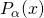, where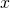is the "truly random" value from some probability space, and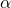is the "fixed but unknown" parameter. Your whole "school of thought" is now focused on clever ad-hoc techniques for computing estimates of this fixed parameter from the provided distribution.

For a pure Bayesian, however, there is no "fixed"that has to be treated somehow separately. Instead,is also a part of some probability space, and instead of writinghe would safely write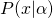,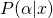, or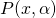. As a result, the probability distribution he works with are not parameterized any more, and all of the clever techniques that the classical statisticians have invented over the centuries for estimating parameters become seemingly useless. At this point a classical statistician puts his hands down and goes home, as there is nothing to do for him - there are no "unknowns". The Bayesian is, however, left to struggle with mathematically trivial, yet computationally incredibly heavy methods for extracting essentially the same values that the classical statistician could have obtained using his "parameter estimation" approaches. That's why the Bayesian "school of thought" is mostly focused on computationally-efficient methods for marginalization and sampling.

In reality, of course, a Bayesian would quite often give up and "cheat", at least partially parameterizing his models and making use of the classical estimation methods, while a "classical" statistician might happen to write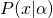and apply the Bayes rule here and there, whenever it seems appropriate. A number of computations derived from the two theoretical backgrounds end up exactly the same.

Thus, in practice, labeling things as "Bayesian" or "non-Bayesian" is still largely a philosophical choice. For example, there are methods in machine learning, ensemble learners, that are somewhy never labeled/marketed as being "Bayesian" nor were they probably invented by someone "Bayesian", although at their core those would be among the best examples of where a Bayesian approach is different from a classical one. Those are also among the best performant models quite often, by the way.

Posted by Konstantin @ 10:04 pm

• ### No Comments

Please note: Comment moderation is enabled and may delay your comment. There is no need to resubmit your comment.

### Calendar

January 2016
M T W T F S S
« Sep   May »
123
45678910
11121314151617
18192021222324
25262728293031